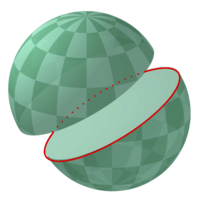# Great circleA great circle divides the sphere in two equal hemispheres

A great circle, also known as an orthodrome or Riemannian circle, of a sphere is the intersection of the sphere and a plane that passes through the center point of the sphere. This partial case of a circle of a sphere is opposed to a small circle, the intersection of the sphere and a plane that does not pass through the center. Any diameter of any great circle coincides with a diameter of the sphere, and therefore all great circles have the same circumference as each other, and have the same center as the sphere. A great circle is the largest circle that can be drawn on any given sphere. Every circle in Euclidean 3-space is a great circle of exactly one sphere.

For most pairs of points on the surface of a sphere, there is a unique great circle through the two points. The exception is a pair of antipodal points, for which there are infinitely many great circles. The minor arc of a great circle between two points is the shortest surface-path between them. In this sense, the minor arc is analogous to “straight lines” in Euclidean geometry. The length of the minor arc of a great circle is taken as the distance between two points on a surface of a sphere in Riemannian geometry. The great circles are the geodesics of the sphere.

In higher dimensions, the great circles on the n-sphere are the intersection of the n-sphere with 2-planes that pass through the origin in the Euclidean space Rn+1.

## Derivation of shortest paths

To prove that the minor arc of a great circle is the shortest path connecting two points on the surface of a sphere, one can apply calculus of variations to it.

Consider the class of all regular paths from a point p to another point q. Introduce spherical coordinates so that p coincides with the north pole. Any curve on the sphere that does not intersect either pole, except possibly at the endpoints, can be parametrized byprovided we allow φ to take on arbitrary real values. The infinitesimal arc length in these coordinates isSo the length of a curve γ from p to q is a functional of the curve given byNote that S[γ] is at least the length of the meridian from p to q:Since the starting point and ending point are fixed, S is minimized if and only if φ' = 0, so the curve must lie on a meridian of the sphere φ = φ0 = constant. In Cartesian coordinates, this iswhich is a plane through the origin, i.e., the center of the sphere.

## Applications

Some examples of great circles on the celestial sphere include the celestial horizon, the celestial equator, and the ecliptic. Great circles are also used as rather accurate approximations of geodesics on the Earth's surface for air or sea navigation (although it is not a perfect sphere), as well as on spheroidal celestial bodies.

The equator of the idealized earth is a great circle and any meridian and its opposite meridian form a great circle. Another great circle is the one that divides the land and water hemispheres. A great circle divides the earth into two hemispheres and if a great circle passes through a point it must pass through its antipodal point.